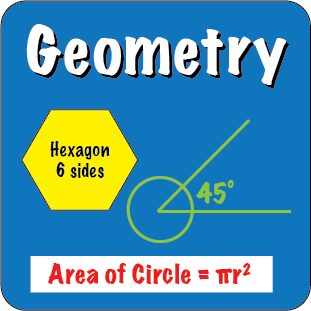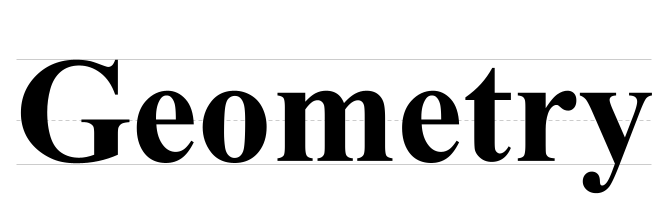Back to Mr. Anker TestsTeachers & Parents: It is recommended that students complete and master
each activity before moving on to the next one.

Basic Geometry
 Basic Geometry 1Basic two dimensional shapes and properties. Basic Geometry 2Work with terms like hexagon, square pyramid, rectangular prism. Basic Geometry 3Work with terms like polygon, right angle, faces, sphere, parallel, segment, etc... Slideshow with LinksStriking Examples of Geometry in Architecture
 Polygon Names 1Basic polygons. Polygon Names 2Features of polygons. Irregular PolygonsFeatures of irregular polygons. The Magic CircleAn Interactive Geometry Overview Lesson (Fun!)
 Parallelograms 1Basic concepts of parallelograms. Parallelograms 2Basic concepts of parallelograms. Parallelograms 3Basic concepts of parallelograms. Magic Circle QuizA quiz based on parts of the 'Magic Circle' Lesson (above)

Area
 Area Set 1Use the formulalength x width = Area. Area Set 2Finding area with whole and half units. Area Set 3Finding the area of complex polygons. Area Set 4Finding the area of more complex polygons.

 Area of Parallelograms 1Use the formulabase x height = Area. Area of Parallelograms 2Use the formulabase x height = Area. Area of Triangles 1Use the formula1/2 base x height = Area. Area of Triangles 2Use the formula1/2 base x height = Area.

Perimeter
 Perimeter 1Calculate area of figures with integer sides. Perimeter 2Calculate area, with emphasis on process and thinking algebraically. Perimeter 3More challenging with an emphasis on thinking algebraically.

Perimeter & Area Using a Ruler
 Finding the Perimeter Using a Ruler 1Refer to rulers to find side lengths & add them. Finding the Perimeter Using a Ruler 2Refer to rulers to find side lengths & add them. Finding the Area Using a Ruler 1Refer to rulers to find the area of a rectangle.

Volume
 Volume 1Use the formula L x W x H = V(cubic units). Volume 2Use the formula L x W x H = V(cubic units).

Linear Measurement
 Measurement 1Measure to the nearest inch, half inch, and quarter inch. Measurement 2Measure to the nearest inch, half inch, and quarter inch. Measurement 3Measure to the nearest whole,half, quarter, and eighth inch. Measurement 4Measure to the nearestsixteenth inch.
 Measurement 5Measure to the nearest centimeter & exact millimeter. Measurement 6Measure & compare with centimeters & millimeters. Measurement 7Measure with fractions of inches, cm & mm, w/ ruler misplaced. Measurement 8Enter measured values for cm, mm, & inches.
 Measurement 9Measure to the nearest centimeter & exact millimeter. Parts of an Inch 1Student recognizes the labeled division of an inch. Parts of an Inch 2Student relies less on the labeling of an inch. Interactive PracticePractice moving the ruler in this activity.

Types of Triangles
 Types of Triangles 1Determine whether a triangle is equilateral, scalene, right, isosceles, etc... Types of Triangles 2Determine whether a triangle is equilateral, scalene, right, isosceles, etc...

Using a Protractor
 Protractor 1Find the degree measure of an angle, as well as naming some angles. Protractor 2Find the degree measure of an angle, as well as naming some angles. Protractor 3Find the degree measure of an angle, as well as naming some angles. Protractor 4Find the degree measure of an angle, as well as naming some angles.

Estimating Angles
 Estimating Angles 1Estimate the degree measure of an angle using a neighboring protractor as a reference. Estimating Angles 2Estimate the degree measure of an angle using a neighboring protractor as a reference. Estimating Angles 3Estimate, by entering a value, the degree measure of an angle, within 10 degrees, using a neighboring protractor as a reference.

Naming Angles
 Name the Angle 1Identify angles as acute, obtuse, right, straight using a protractor as reference. Name the Angle 2Identify angles as acute, obtuse, right, straight w/ & w/o a protractor as reference. Name the Angle 3Identify angles as acute, obtuse, right, straight w/o using a protractor as reference. Name the Angle 4Identify angles as acute, obtuse, right, straight w/o using a protractor as reference.

Calculating Angles
 A Triangle's Missing Angle 1Using addition or subtraction, determine the angle measure of an unlabeled angle in a triangle. A Triangle's Missing Angle 2Using addition or subtraction, determine the angle measure on af unlabeled angle in a triangle. A Quadrilateral's Missing Angle 1Using addition or subtraction, determine the angle measure of an unlabeled angle in a quadrilateral. A Quadrilateral's Missing Angle 2Using addition or subtraction, determine the angle measure of an unlabeled angle in a quadrilateral.

The activities below will be ready soon.
 CongruencyIdentify congruent figures. SymmetryIdentify figures with a line of symmetry. Types of Angles 3Finding Complements & Supplements to given angles. Types of Angles 4Finding Complements & Supplements to given angles.